Home | | Transforms and Partial Differential Equations | Z-Transforms and Difference Equations

# Z-Transforms and Difference Equations

1 Introduction 2 Linear Difference Equations 3 Z-Transforms And Its Properties 4 Inverse Z-Transforms 5 Convolution Theorem 6 Applications Of Z-Transforms To Difference Equations 7 Formation Of Difference Equations

Z-Transforms AND DIFFERENCE EQUATIONS

1 INTRODUCTION

2 LINEAR DIFFERENCE EQUATIONS

3 Z-TRANSFORMS AND ITS PROPERTIES

4 INVERSE Z-TRANSFORMS

5 CONVOLUTION THEOREM

6 APPLICATIONS OF Z-TRANSFORMS TO DIFFERENCE EQUATIONS

7 FORMATION OF DIFFERENCE EQUATIONS

1 Introduction

The Z-transform plays a vital role in the field of communication Engineering and control Engineering, especially in digital signal processing. Laplace transform and Fourier transform are the most effective tools in the study of continuous time signals, where as Z –transform is used in discrete time signal analysis. The application of Z – transform in discrete analysis is similar to that of the Laplace transform in continuous systems. Moreover, Z-transform has many properties similar to those of the Laplace transform. But, the main difference is Z-transform operates only on sequences of the discrete integer-valued arguments. This chapter gives concrete ideas about Z-transforms and their properties. The last section applies Z-transforms to the solution of difference equations.

Difference Equations

Difference equations arise naturally in all situations in which sequential relation exists at various discrete values of the independent variables. These equations may be thought of as the discrete counterparts of the differential equations. Z-transform is a very useful tool to solve these equations.

A difference equation is a relation between the independent variable, the dependent variable and the successive differences of the dependent variable.are difference equations.

The differences Dyn, D2yn, etc can also be expressed as.

Dyn =  yn+1 - yn,

D2yn = yn+2 - 2yn+1 + yn.

D3yn = yn+3 - 3yn+2 + 3yn+1 - yn  and so on.

Substituting these in (i) and (ii), the equations take the formNote that the above equations are free of Ds¢.

If a difference equation is written in the form free of Ds,¢then the order of   difference equation is the difference between the highest and lowest subscripts of y‟s occurring in it. For example, the order of equation (iii) is 2 and equation (iv) is 1.

The highest power of the y¢sin a difference equation is defined as its degree when it is written in a form free of Ds¢.For example, the degree of the equations yn+3 + 5yn+2 + yn = n2 + n + 1 is 3 and y3 n+3 + 2yn+1 yn = 5 is 2.

2 Linear Difference Equations

A linear difference equation with constant coefficients is of the form

a0 yn+r + a1 yn+r -1 + a2 yn+r -2 + . . . . +aryn = f(n).

i.e., (a0Er + a1Er-1 + a2 Er-2 + . . . . + ar)yn = f(n)   ------(1)

where a0,a1, a2, . . . . . ar  are constants and f(n) are known functions of n.

The equation (1) can be expressed in symbolic form as

f(E) yn = f(n) ----------(2)

If  f(n) is zero, then equation (2) reduces to

f (E) yn = 0 ----------(3)

which is known as the homogeneous difference equation corresponding to (2).The solution of (2) consists of two parts, namely, the complementary function and the particular integral.

The solution of equation (3) which involves as many arbitrary constants as the order of the equation is called the complementary function. The particular integral is a particular solution of equation(1) and it is a function of „n‟ without any arbitrary   constants.

Thus the complete solution of (1) is given by  yn = C.F + P.I.

Example 1

Form the difference equation for the Fibonacci sequence .

The integers 0,1,1,2,3,5,8,13,21, . . . are said to form a Fibonacci sequence.

If yn be the nth term of this sequence, then

yn = yn-1 + yn-2 for n > 2

or  yn+2 - yn+1 - yn = 0 for n > 0

3 Z - Transforms and its Properties

Definition

Let {fn} be a sequence defined for n = 0,1,2,…….,then its Z-transform F(z) is defined aswhenever the series converges and it depends on the sequence {fn}.

The inverse Z-transform of F(z) is given by Z-1{F(z)} = {fn}.

Note: If {fn}   is   defined   for   n   =   0,   ±   1,   ±   2,   …….,Properties of Z-Transforms

1.     The Z-transform is linear.

i.e,  if F(z) = Z{fn} and G(z) = Z{gn}, then

Z{afn + bgn} = aF(z) + bG(z).4. If Z{fn} = F(z), then

Z{fn+k}= zk{ F(z) –f0 –(f 1 / z ) - … - ( fk-1 / zk-1) } (k > 0)

ProofIn Particular,

(i) Z{f n+1} = z {F(z) - f0}

(ii) Z{f n+2}= z2 { F(z) –f0 –(f1/z) }

Corollary

If  Z{fn} = F(z), then Z{fn–k} = z-k F(z).

(5) Initial value TheoremProof

We know that F (z) = f0 + f1 z-1 + f2z-2 + . . .

Taking limits as z ® ¥on both sides, we get(6) Final value TheoremSOME STANDARD RESULTS

1.                 Z{an} = z / (z-a), for |z| > |a|.

Proof

By definition, we haveIn particular, we have

Z{1} = z / (z-1), (taking a = 1).

and      Z{(-1)n} = z / (z +1), (taking a = -1).

2.  Z{nan} =  az /(z-a)2

Proof: By property, we haveSimilarly, we can prove

Z{n2an} = {az(z+a)}/ (z-a)3Equating the Real and Imaginary parts, we getEquating the Real and Imaginary parts, we get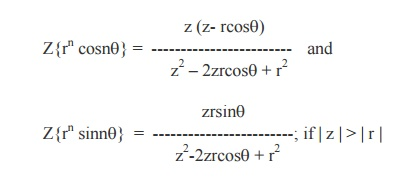Table of Z –TransformsExample 2

Find the Z–transform of

1.     n(n-1)

2.     n2 + 7n + 4

3.     (1/2)( n+1)(n+2)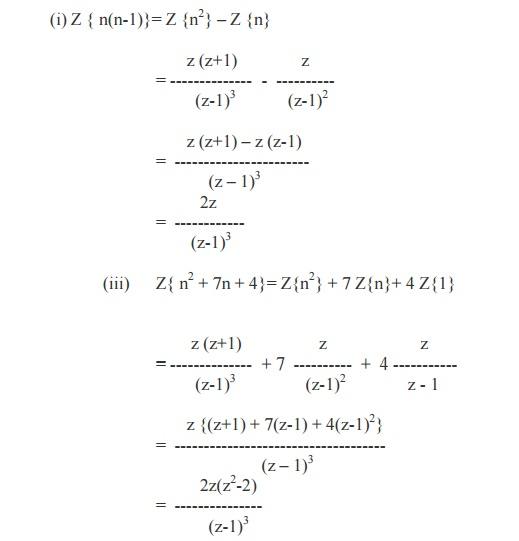Example 3

Find the Z- transforms of 1/n   and  1/n(n+1)= - log (1 –1/z ) if  |1/z| < 1

= - log (z-1 / z)

=   log (z/z-1), if | z | >1.Example 4

Find the Z- transforms of

(i) cos np/2

(ii)   sin np/2Example 5

Show that Z{1/ n!} = e1/z and hence find Z{1/ (n+1)!} and  Z{1/ (n+2)!}We know that Z{fn+1} = z { F(z) –f0}

Therefore,Example 6

Find the Z- transforms of the following4 Inverse Z –Transforms

The inverse Z –transforms can be obtained by using any one of the following methods.They are

I.Power series method

II. Partial fraction method

III.  Inversion Integral method

IV.  Long division method

I. Power series method

This is the simplest method of finding inverse Z –transform. Here F(z) can the be expanded in a series of ascending powers  and the coefficient of z –n will be the of z desired inverse Z- transform.

Example 8

Find the inverse Z –transform of log {z /(z+1)} by power series method.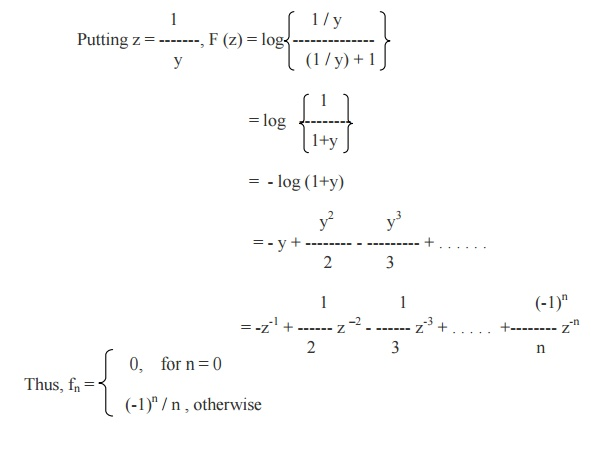II. Partial Fraction Method

Here, F(z) is resolved into partial fractions and the inverse transform can be taken directly.

Example 9

Find the inverse Z –transform of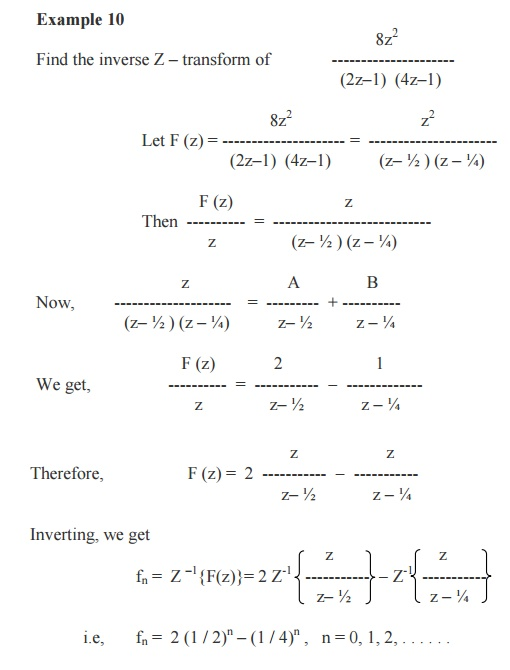Inversion Integral Method or Residue Method

The inverse Z-transform of F (z) is given by the formula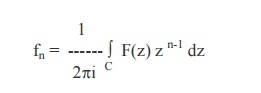Sum of residues of F(z).zn-1 at the poles of F(z) inside the contour C which is drawn according to the given Region of convergence.

Example 12

Using the inversion integral method, find the inverse Z-transform ofIts poles are z = 1,2 which are simple poles.

By inversion integral method, we have\Sum of Residues = -3 + 3.2n = 3 (2n-1).

Thus the required inverse Z-transform is

fn = 3(2n-1),   n   =   0,   1,   2,   …

Example 13

Find the inverse z-transform ofThe pole of F(z) is z = 1, which is a pole of order 3. By Residue method, we haveIV. Long Division Method

If F(z) is expressed as a ratio of two polynomials, namely, F(z) = g(z-1) / h(z-1), which can not be factorized, then divide the numerator by the denominator and the inverse transform can be taken term by term in the quotient.

Example 14Thus F(z) = 1 + 3z-1 + 3z-2 + 3z-3 + . . . . . .

Now, Comparing the quotient withExample 15

Find the inverse Z-transform of5 CONVOLUTION THEOREM

If  Z-1{F (z)} = fn  and  Z-1{G(z)} = gn, thenExample 16

Use convolution theorem to evaluateWe know that Z-1 {F(z). G(z)} = fn*gn.Example 18

Use convolution theorem to find the inverse Z- transform6 Application of Z - transform to Difference equations

As we know, the Laplace transforms method is quite effective in solving linear differential equations, the Z - transform is useful tool in solving linear difference equations.

To solve a difference equation, we have to take the Z - transform of both sides of the difference equation using the property

Z{fn+k}= zk{ F(z) –f0 –(f 1 / z ) - … - ( fk-1 / zk-1) } (k > 0)

Using the initial conditions, we get an algebraic equation of the form F(z) = f(z).

By taking the inverse Z-transform, we get the required solution fn of the given difference equation.

Exmaple 19

Solve the difference equation  yn+1 + yn = 1, y0 = 0, by Z - transform method.

Given equation is yn+1 + yn = 1                         ---------- (1)

Let Y(z) be the Z -transform of {yn}.

Taking the Z - transforms of both sides of (1), we get

Z{yn+1} +  Z{yn} = Z{1}.

ie, z {Y(z) - y0} + Y(z) = z /(z-1).

Using the given condition, it reduces toOn taking inverse Z-transforms, we obtain

yn = (1/2){1 - (-1)n}

Example 20

Solve         yn+2       + yn = 1, y0 = y1 = 0 ,     using Z-transforms.

Consider      yn+2       + yn = 1   -------------       (1)

Taking Z- transforms on both sides, we get

Z{yn+2}+ Z{yn} = Z{1}Using Inverse Z-transform, we get

yn =(½){1 - cos (np/ 2) - sin (np/ 2)}.

Example 21

Solve yn+2 + 6yn+1 + 9yn = 2n,  y0 = y1 = 0, using Z-transforms.

Consider yn+2 + 6yn+1 + 9yn = 2n -------- (1)

Taking the Z-transform of both sides, we get

Z{yn+2} + 6Z{yn+1} + 9Z{yn} = Z {2n}On taking Inverse Z-transforms, we get

yn = (1/ 25){ 2n - (-3)n + (5/3) n (-3)n}.

Example 22

Solve the simultaneous equations

xn+1 - yn = 1; yn+1 - xn = 1 with x (0) = 0; y (0) = 0.

The given equations are

xn+1 - yn = 1, x0 = 0

yn+1 - xn  =1,

y0 = 0   -------------- (2)

Taking Z-transforms, we getOn taking the inverse Z-transform of both sides, we have xn = n and yn = n , which is the required solution of the simultaneous difference equations.

Example 23

Solve xn+1 = 7xn + 10yn ;  yn+1 = xn + 4yn, with x0 = 3, y0 = 2

Given

xn+1 = 7xn + 10yn------------- (1)

yn+1 = xn + 4yn------------- (2)

Taking Z- transforms of equation(1), we get

z { X(z) - x0} = 7 X(z) + 10 Y(z)

(z - 7) X(z) –10 Y(z) = 3z ----------(3)

Again taking Z- transforms of equation(2), we get

z {Y(z) - y0} = X(z) + 4Y(z)

-X(z) + (z - 4)Y(z) = 2z ----------        (4)

Eliminating „x‟   from   (3)   &   (4),   we   getTaking Inverse Z-transforms, we get

yn = 9n + 2n.

From (2), xn = yn+1 - 4yn  = 9n+1 + 2n+1 - 4 (9n + 2n)

= 9.9n + 2.2n - 4.9n - 4.2n

Therfore, xn = 5.9n - 2.2n

Hence the solution is  xn = 5.9n - 2.2n   and  yn = 9n + 2n.

Exercises

Solve the following difference equations by Z –transform method

1. yn+2 + 2yn+1 + yn = n,  y0 = y1 = 0

2. yn+2 –yn = 2n, y0 = 0, y1 = 1

3. un+2 –2cosaun+1+ un=0, u0 = 1, u1 = cosa

4. un+2 = un+1 + un, u0 = 0, u1 = 1

5. yn+2 –5yn+1+ 6yn = n (n-1), y0 = 0, y1 = 0

6. yn+3 –6yn+2 + 12yn+1 –8yn = 0, y0 = -1, y1 = 0, y2 = 1

7 FORMATION OF DIFFERENCE EQUATIONS

Example

Form the difference equation16 y n -4 yn+2 =0

-4( y n +2 -4yn ) =0

y n +2 -4 yn =0

Exercise:

1.      Derive the difference equation form yn =( A +Bn)( 3)-n

2.      Derive the difference equation form U n =A2n +Bn

Study Material, Lecturing Notes, Assignment, Reference, Wiki description explanation, brief detail
Mathematics (maths) : Z-Transforms and Difference Equations : Z-Transforms and Difference Equations |